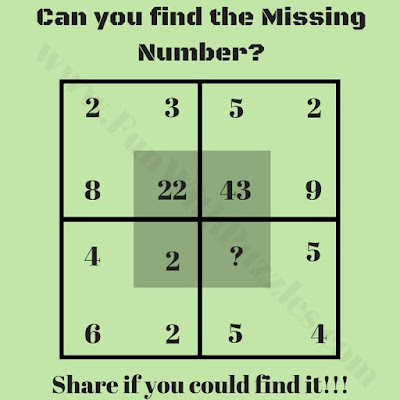## Tuesday, September 22, 2020

This Brain Maths Question is for school going kids. In this Maths Puzzle Question, there are some numbers given in four squares. Numbers in each of the square relates to each other Mathematically. Can you decipher this Mathematical relationship or pattern among the numbers in each of the squares and then find the missing number which will replace the question mark in one of the squares?Can you find the missing number?

The answer to this "Brain Maths Question for Students", can be viewed by clicking the below button.

1.1.